## Infinite Limits

Some functions “take off” in the positive or negative direction (increase or decrease without bound) near certain values for the independent variable. When this occurs, the function is said to have an infinite limit; hence, you write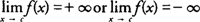. Note also that the function has a vertical asymptote at x = c if either of the above limits hold true.

In general, a fractional function will have an infinite limit if the limit of the denominator is zero and the limit of the numerator is not zero. The sign of the infinite limit is determined by the sign of the quotient of the numerator and the denominator at values close to the number that the independent variable is approaching.

Example 1: Evaluate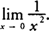.

As x approaches 0, the numerator is always positive and the denominator approaches 0 and is always positive; hence, the function increases without bound and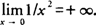. The function has a vertical asymptote at x = 0 (see Figure ).Figure 1 The graph of y = 1/ x 2.

Example 2: Evaluate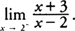.

As x approaches 2 from the left, the numerator approaches 5, and the denominator approaches 0 through negative values; hence, the function decreases without bound and. The function has a vertical asymptote at x = 2.

Example 3: Evaluate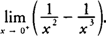.

Rewriting 1/ x 2 − 1/ x 3 as an equivalent fractional expression ( x − 1)/ x 3, the numerator approaches −1, and the denominator approaches 0 through positive values as x approaches 0 from the right; hence, the function decreases without bound and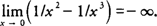. The function has a vertical asymptote at x = 0.

A word of caution: Do not evaluate the limits individually and subtract because ±∞ are not real numbers. Using this example,which is meaningless.

Back to Top
A18ACD436D5A3997E3DA2573E3FD792A